## Wednesday, September 26, 2012

### Linearization

In http://yetanothermathprogrammingconsultant.blogspot.com/2012/09/bi-criteria-optimization.html I discussed an algorithm to trace the efficient frontier. We solve a number of non-convex MINLP’s for this. It is fairly easy to linearize the quadratic function by observing that the zk's only assume discrete values 0,1,..,cp.I used something like:

The MIP formulation seems to perform a little bit better even though the model size (in terms of variables and equations) increases significantly: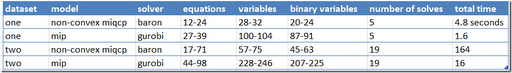Note: the size of the problems increases for each new non-dominated point. We give here the smallest (i.e. first) problem size and the largest (i.e. last) one.

## Monday, September 24, 2012

### Bi-criteria optimization

In the following paper http://nvlpubs.nist.gov/nistpubs/jres/111/2/V111.N02.A05.pdf a two objective model is developed. The usual way I see such models implemented is by adding weights in some linear fashion, e.g.:Here a somewhat unusual form is used: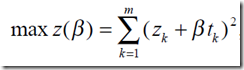Indeed when β=0 we get a single objective scheduling problem:Here the slack zk in bin k is maximized using a quadratic objective. This is not a trivial problem to solve in itself as the problem is non-convex and we need a global solver. The authors use Lingo but other suitable solvers are Baron and GloMiQo. (Looking at the data – all integer – it should not be too difficult to linearize this at the expense of extra binary variables).

When we look at the 2-objective problem formulation we can observe this can be written as:When β is fixed the last term is constant, so we are left with: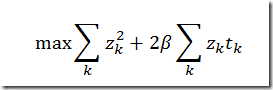Note that the paper want to maximize the slacks zk, but minimize the start time xi,ktk. I did not immediately understand how this objective can actually work. The more direct approach I would use is: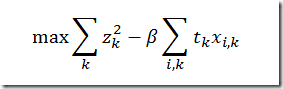or may be: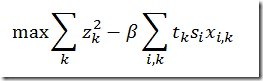Actually what is proposed in the paper is quite clever. Observe that minimizing the tksixi,k's is equivalent to maximizing tkzk (as the z is a slack indicating unused capacity).

The paper indicates it finds three different solutions. They probably use a scheme trying beta=0,0.01,0.02,.., or may be some bisection. There is a much better approach where we solve a single problem for each non-dominated point (plus one problem to detect there are no more such points). Using the separable formulation with: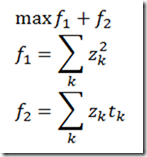we can add some equations that allow us to trace the efficient frontier. This actually gives us 4 non-dominated solutions: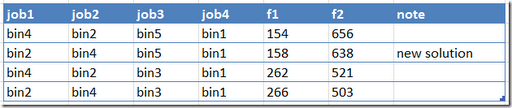For a second data set the paper reports 8 different solutions. My model generates 18 non-dominated solutions: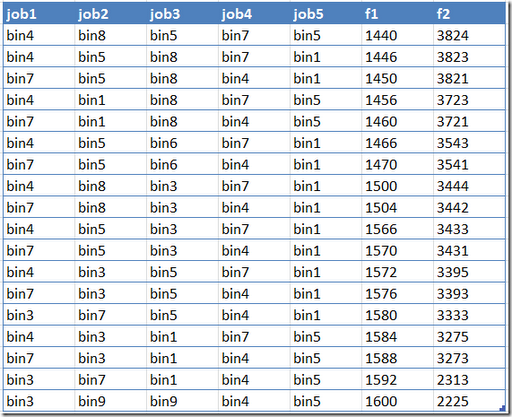My conclusion: although the proposed formulation is quite clever, a more traditional formulation allows us to better trace the efficient frontier.

## Friday, September 21, 2012

### Browser compatibility

I am a bit surprised that now browsers are around for so long, that we still see incompatibilities w.r.t. JavaScript and HTML/DOM. I had some code that worked fine for IE and Chrome. Turns out Firefox did not work. Here is the reason: http://stackoverflow.com/questions/1359469/innertext-works-in-ie-but-not-in-firefox.

I used the fix from http://blog.coderlab.us/2006/04/18/the-textcontent-and-innertext-properties/.

## Wednesday, September 12, 2012

### Preemptive jobs

When doing some background research on a scheduling problem I was looking at the paper http://www.ijbssnet.com/journals/Vol_3_No_4_Special_Issue_February_2012/15.pdf. The model formulation is: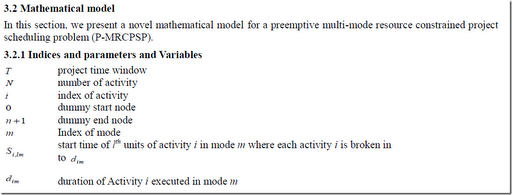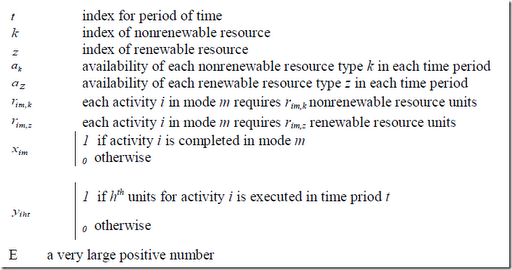The first minor issue is notational. I suspect si,lm should really be variable with three indices: si,l,m. I am unsure why the second and third indices are concatenated. In equations (2)-(5) we see that also a 2 index version is used.

The second problem I see is equation (6). I suspect this should read: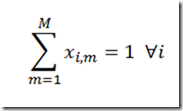Equation (7) does not look correct to me either. Could be they really mean:

Equation (10) introduces suddenly a xi,j,m which probably just should read xi,m. This equation is nonlinear as presented but probably can be linearized easily. In general the capacity constraint for renewable resources is indexed by t, but here in eq (10) the authors propose to sum over t. This cannot be correct either.

To make things worse, the presented solution is of the small sample problem is incorrect. They show: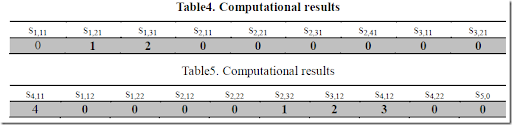When we look at s(2,v,2) we see:

 job part mode start time 2 1 2 0 2 2 2 0 2 3 2 1

I.e. part 1 and 2 are executed in the same time slot.

This is a small model but these typos do not make it very easy to reproduce results.

Update: similar mistakes are found in an earlier paper: www.journal-archieves14.webs.com/636-642.pdf. So these are not just typos. I suspect the model was done in Excel, for the following reasons:

• Excel models are always very messy
• they are often difficult to translate one-to-one to a well formulated mathematical model
• Excel handles non-linear models
• Excel has a 2d grid, which may explain there preference for 2d variables.

When developing a non-trivial model it is often a good idea to use a modeling language such as AMPL or GAMS, as this in sense will check your formulation. The above mistakes would probably not have been made in that case. Excel provides very limited support for more complex models, and from experience I can say Excel models are difficult to understand and debug. This may well be another piece of support for this.

Anyway, this is a good example how not to publish models. Of course in journals with better reviewers a paper like this would probably not have been accepted. The presented model equations and results are incorrect.

My solution looks like: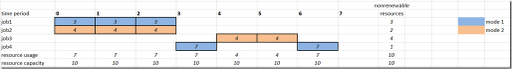The model has no penalty on preemption, so there is no incentive to make jobs as much as possible uninterrupted.